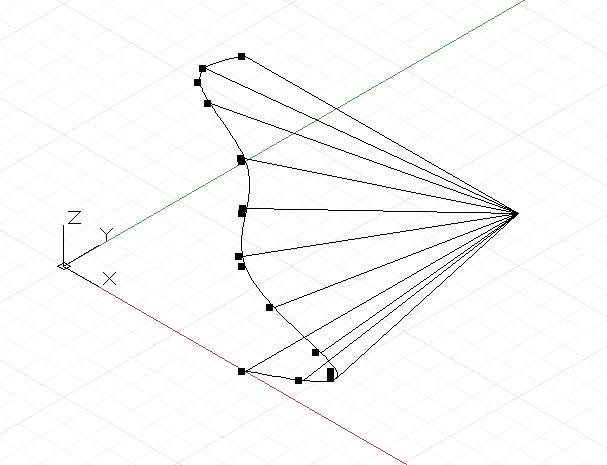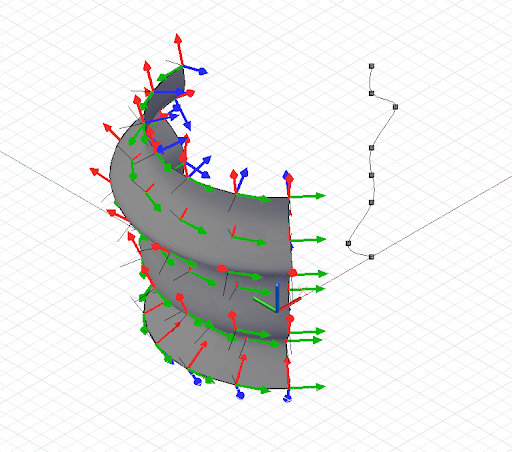# 幾何參數化

``````pts = [ [p1, p2, p3],
[p4, p5, p6],
[p7, p8, p9] ];
``````

p1 會有 u = 0 v = 0 的參數，p9 會有 u = 1 v = 1 的參數。``````pts = {};
pts = Point.ByCoordinates(4, 0, 0);
pts = Point.ByCoordinates(6, 0, 1);
pts = Point.ByCoordinates(4, 0, 2);
pts = Point.ByCoordinates(4, 0, 3);
pts = Point.ByCoordinates(4, 0, 4);
pts = Point.ByCoordinates(3, 0, 5);
pts = Point.ByCoordinates(4, 0, 6);

crv = NurbsCurve.ByPoints(pts);

pts_at_param = crv.PointAtParameter(0..1..#11);

// draw Lines to help visualize the points
lines = Line.ByStartPointEndPoint(pts_at_param,
Point.ByCoordinates(4, 6, 0));
````````````pts = {};
pts = Point.ByCoordinates(4, 0, 0);
pts = Point.ByCoordinates(3, 0, 1);
pts = Point.ByCoordinates(4, 0, 2);
pts = Point.ByCoordinates(4, 0, 3);
pts = Point.ByCoordinates(4, 0, 4);
pts = Point.ByCoordinates(5, 0, 5);
pts = Point.ByCoordinates(4, 0, 6);
pts = Point.ByCoordinates(4, 0, 7);

crv = NurbsCurve.ByPoints(pts);

axis_origin = Point.ByCoordinates(0, 0, 0);
axis = Vector.ByCoordinates(0, 0, 1);

surf = Surface.ByRevolve(crv, axis_origin, axis, 90,
140);

cs_array = surf.CoordinateSystemAtParameter(
(0..1..#7)<1>, (0..1..#7)<2>);

def make_line(cs : CoordinateSystem) {
lines_start = cs.Origin;
lines_end = cs.Origin.Translate(cs.ZAxis, -0.75);

return = Line.ByStartPointEndPoint(lines_start,
lines_end);
}

lines = make_line(Flatten(cs_array));
``````